# 10.95. Kirby Monetary Choice Questionnaire (MCQ)¶

Series of hypothetical choices pitting an immediate amount of money against a delayed amount of money, to measure delay discounting.

## 10.95.1. History¶

• Kirby et al. (1999) [27-item version].

• Based on the earlier 21-item version: Kirby & Maraković (1996).

## 10.95.2. Source¶

• From Kirby et al. (1999) as above.

## 10.95.3. Calculations¶

Hyperbolic delay discounting. The principle is to judge the value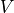of two options,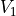and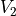. Each has an amount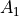,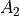and a delay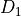,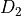. Hyperbolic delay discounting is assumed, according to the discounting parameter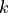: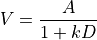In the case of the Kirby MCQ, one option has zero delay (the small immediate reward or SIR – call it option 1):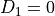. One has a delay (the large delayed reward or LDR – call it option 2).

The values are therefore: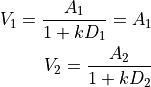At indifference: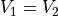so at indifference: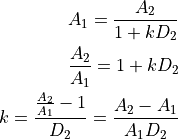Given a set of trials, a subject’s indifference pointcan be calculated in various ways.

Kirby’s method (Kirby et al. 1999; Kirby, 2000). For each question, at indifference,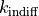can be calculated from the equations above via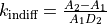. Kirby’s method compares subject’s choices against these indifferencevalues: if the subject chooses the delayed option, then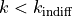, and if the subject chooses the immediate option, then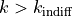. For a consistent series of choices, the geometric mean of the testedvalues straddling the subject’s indifference point is taken as the subject’svalue. If the subject’svalue falls outside the tested range (i.e. all choices were for the immediate, or all for the delayed, option), then the most extreme assessedis used. Where choices are not consistent, then the subject’s value ofis taken as thevalue with which the subject’s choices were most consistent (i.e. the most choices were as predicted by that value of) or, if there is no clear winner, the geometric mean of multiple possible testedvalues that were equally and maximally consistent with the subject’s choice. This geometric mean approach reduces to the simple ‘consistent’ approach for consistent choices, and thus may be used throughout.

Wileyto et al.’s method (Wileyto et al., 2004). This method defines the reward ratio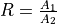, and then predicts the probability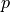of choosing the delayed response using a logistic regression,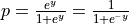where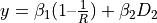. That is,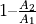andare used as predictors, obtaining the coefficients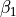and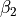. At indifference, where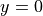, this gives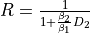, and henceis estimated by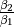. However, it is possible for such estimates to be negative under conditions of inconsistent choice.

Todo

Reference RNC new method when published.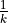is the delay at which a prospective reward has decayed to half its original value.

## 10.95.4. Intellectual property rights¶

Believed to contain no significant intellectual property, aside from the code, which is part of CamCOPS.